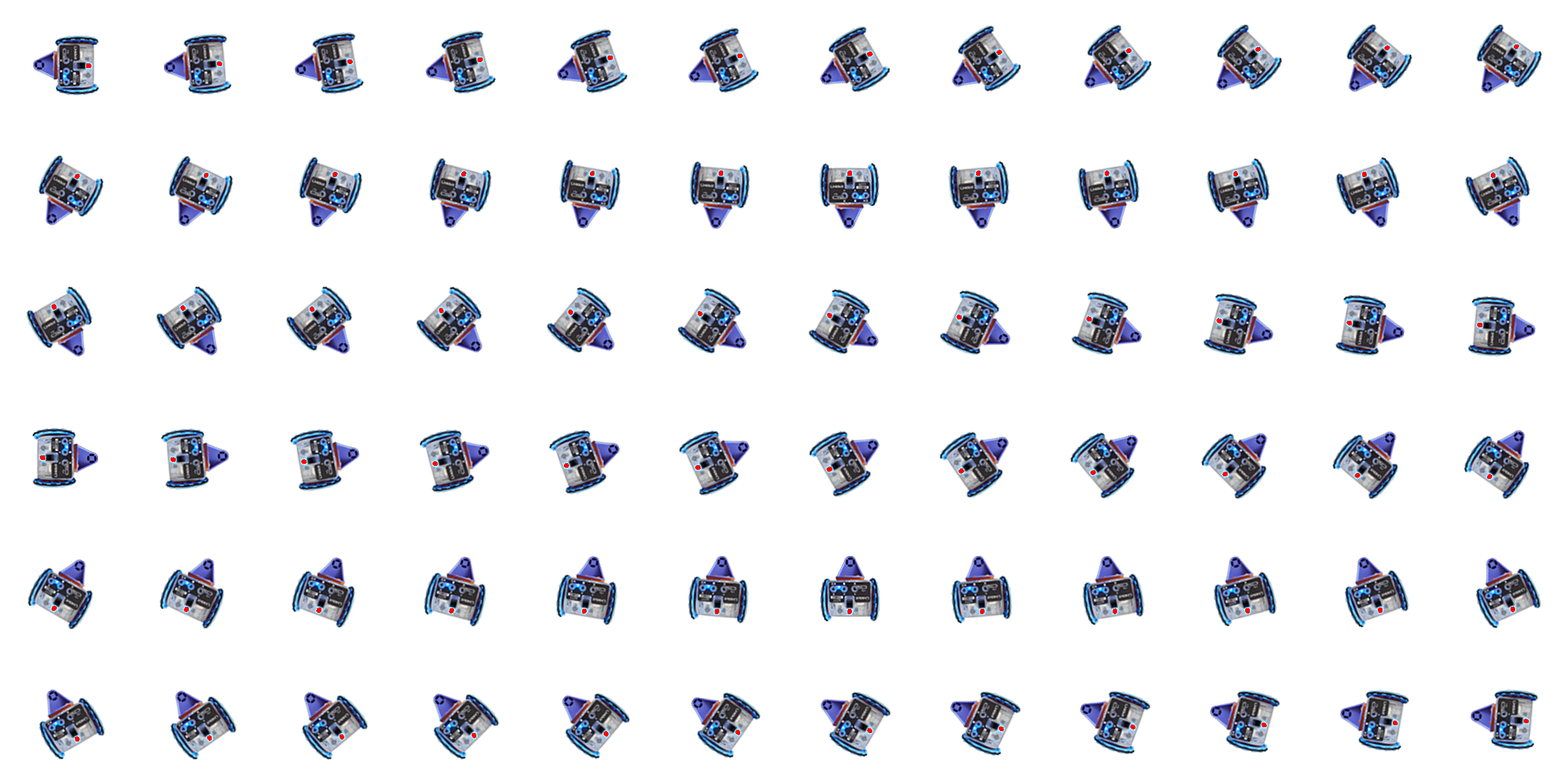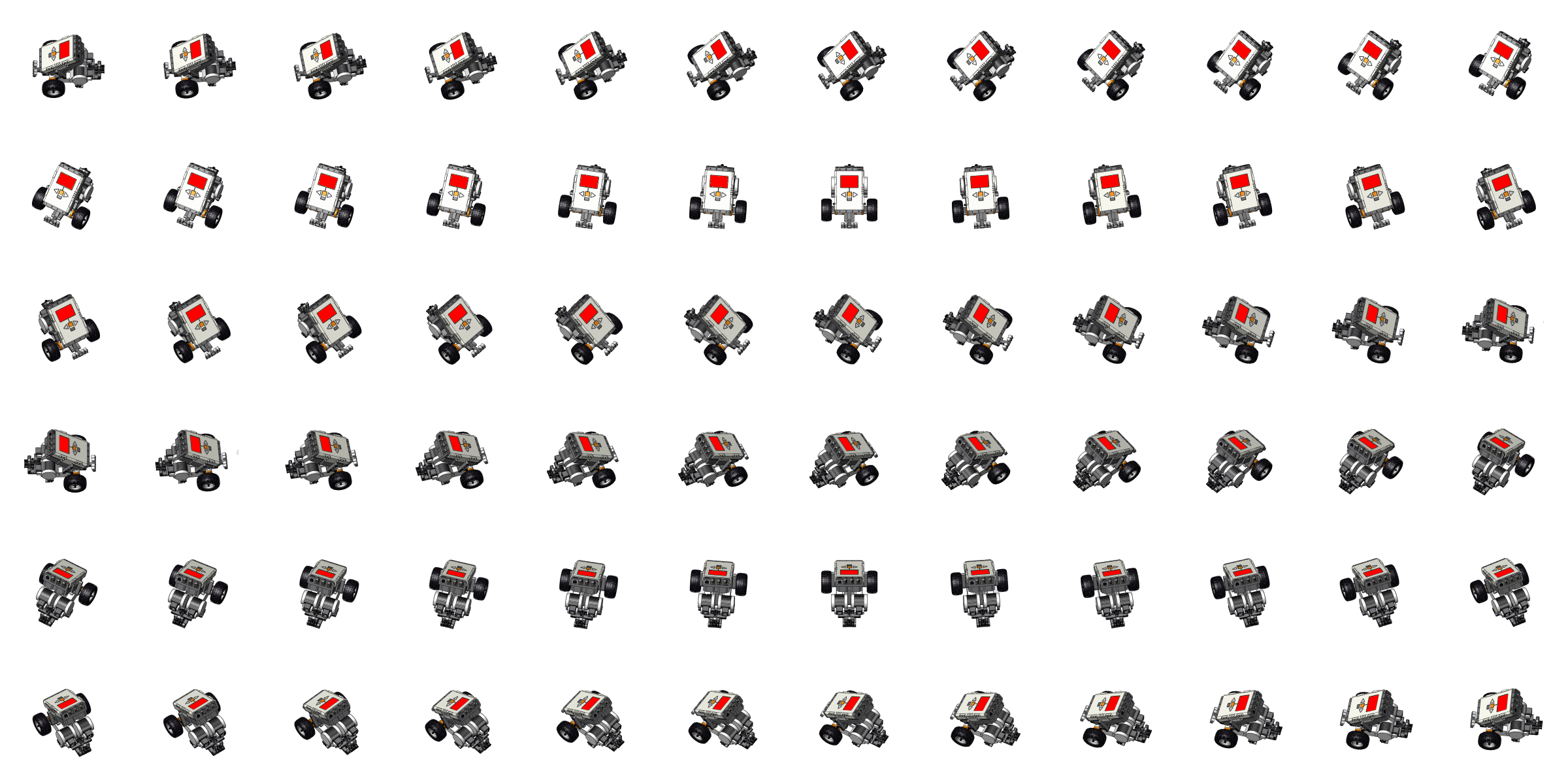Learning Math with Coding and RoboticsGrid Size: S M L Simple View: MinView:
Determining Relationships Using Student Homework Data
 A math teacher decides to divide her class in to two groups and record a score for each student’s satisfaction based on the number of homework assignments assigned. She assigns students who claim to enjoy math to Group 1, and students who claim to dislike math to Group 2. Run the program, and use the scatter plot in the grid to determine the relationship between the number of homework assignments and student satisfaction scores of each group.
 Piano Symbol Word APCSP Hardware LArduino Workspace Load Save Ch Ch Shell Console
Lock
roboblockyA411.chTime